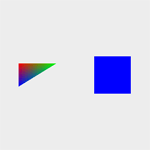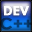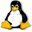Home Latest News Archive Tutorials C++ 2 3 4 Win32 Java OpenGL GLUT OpenGL ES 2 3 4 DirectX VB.NET HTML Photoshop 3dsmax Forums Projects Modelling Links About Us Search Recent Tutorials C / C++ Win32 OpenGL OpenGL ES GLUT## Transformations

Tutorials > OpenGL > Transformations

### IntroductionYou may find yourself needing to transform your primitives in various ways.

OpenGL provides 3 ways of transforming your primitives :

1. Scaling
2. Translation
3. Rotation

Scaling will enlarge or reduce the size of your primitives. Translation will move your primitives across the 3 axes in 3D space. Rotation obviously rotates your object along the 3 axes.

### What else will be covered?

To show the effect of rotation, we will be introducing you to animation in this tutorial.

Contents of main.cpp :

At the end of this tutorial, you will have one triangle and one quad which are rotating around both the x and y axes. We therefore need to create 2 variables to hold the current rotation values.

```float xrot = 0.0f;
float yrot = 0.0f;
```

We will be rendering a triangle on the left-hand side of the screen and a square on the right-hand side. The triangle will be smooth shaded and the square will be flat shaded.

```void display()
{
glClear(GL_COLOR_BUFFER_BIT);
```

Our transformation code follows. Remember in the reshape function that we set the modelview matrix as the current matrix. This matrix is now used for transformations. The 3 transformations are accomplished by using the glTranslatef, glScalef and glRotatef functions. The f at the end of the function indicates that all parameters must be floats. You could also place a d instead to require GLdouble variables. Many OpenGL functions are used in this way. Sometimes a v is also added to indicate that an array is being passed. We already saw similar conventions used with the glColor functions.

After rendering our triangle, we do not want all other shapes that will be rendered afterwards to also be affected by the transformations. The glPushMatrix and glPopMatrix functions can be used to push and pop the matrix onto and off of the stack. You can place your transformation code between these two functions to make sure that the modelview matrix is reset to its original value after the code segment.

```	// TRIANGLE
glPushMatrix();
```

We now move onto our 3 transformations. Each successive transformation affects the modelview matrix. Note that the order in which you place the transformation affects the overall result as matrix multiplication is not the same when reversed.

If you translate something to the right and then rotate it, you will view a rotated shape sitting on the horizontal axis. If you first rotate the object and then translate it, you will view a rotated shape that has been moved out diagonally from the origin. The result of transformations appear in reverse i.e. if you do a translate and then a scale, the object will first be scaled and then translated. Try changing the order and values of the functions below to see what affect each one has.

We pass 3 floats to the glTranslatef function, specifying the value to move the drawn objects in the x, y and z direction. Our first transformation is to move the triangle right 0.25 units and up 0.5 units.

```		glTranslatef(0.25f, 0.5f, 0.0f);
```

Our next transformation scales the object. Remember we said that the object must be centered on the origin. This is because the scale transformation takes all 3 values of each vertex and multiplies them by the parameters passed onto the glScalef function. If the object was placed with its bottom-left corner on the origin, the scale transformation would cause the object to reduce in size but the bottom-left corner would remain on the origin.

The code below reduces the size of the triangle by half. Note that you can scale an object by a different amount in each axis.

```		glScalef(0.5f, 0.5f, 0.5f);
```

Our rotation transformation is done below. The first parameter specifies the angle in degrees to rotate the objects.

The next 3 parameters are used to specify in what axis to rotate. These specify a vector. A value of 1.0 is normally used to specify an axis. You can think of rotation along the x axis being similar to a car tilting up and down on a hill with you looking forward. Rotation along the y axis is similar to how a drill bit rotates when you are drilling into a piece of wood. Rotation along the z axis is similar to a fan's rotation when looking at the blades head-on.

```		glRotatef(xrot, 1.0f, 0.0f, 0.0f);
```

We then draw the triangle as per normal. The triangle will appear at half its size on the left-hand side of the screen. Each vertex is given a different color as in previous tutorials.

```		glBegin(GL_TRIANGLES);
glColor3f(1.0f, 0.0f, 0.0f);
glVertex3f(-0.25f, -0.25f, 0.0f);
glColor3f(0.0f, 1.0f, 0.0f);
glVertex3f( 0.25f, -0.25f, 0.0f);
glColor3f(0.0f, 0.0f, 1.0f);
glVertex3f(-0.25f,  0.25f, 0.0f);
glEnd();
```

The current matrix is now popped off to revert back to the original matrix for further rendering.

```	glPopMatrix();
```

The transformations made for the square are the same except that it is moved 0.75 units to the right instead of 0.25. The shape is also rotated along the y axis instead of the x axis. A single blue color is specified before the vertices to result in a flat-shaded primitive. Note we do not need to change the shading mode as we are only specifying one color.

```	// SQUARE
glPushMatrix();

glTranslatef(0.75f, 0.5f ,0.0f);
glScalef(0.5f, 0.5f, 0.5f);
glRotatef(yrot, 0.0f, 1.0f, 0.0f);

glColor3f(0.0f, 0.0f, 1.0f);
glVertex2f(-0.25f, -0.25f);
glVertex2f( 0.25f, -0.25f);
glVertex2f( 0.25f,  0.25f);
glVertex2f(-0.25f,  0.25f);
glEnd();

glPopMatrix();

glFlush();
}
```

The last step is to edit the idle function. Here, we simply increase the angle variables defined at the beginning of the program. If you are using GLUT, you will also need to make a call to glutPostRedisplay, causing the window to be redisplayed.

```void idle()
{
xrot += 0.2f;
yrot += 0.3f;
}
```

Well done. You should now be able to transform your primitives and should also be able to create simple animation.

Please let me know of any comments you may have : Contact Me

 Win32 Source Files :GLUT Source Files :Last Updated : 14 October 2005

 < Tutorial 09 - Window Resizing Tutorial 11 - Depth >